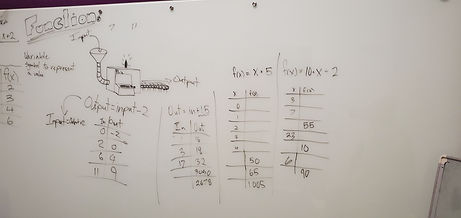Mr. Jake

Target 1​

Lesson Type:

New

Algebra

:

Function

Use input and output data to determine a function equation.

1:

Understand that a function is a rule that gives one output to each input.

2:

Understand that by studying the proportional relationship between inputs and outputs, a function (equation) can be derived.

3:

Use a function equation to fill out a function table.

6th

Vocabulary:

Function, Variable, Input, Output

Activities:

Given function equations, filled out an input/output tableHome Exploration

Guiding Questions:Absent Students:

Target 2

:

1:

Understand that angles are measured in degrees (from 0 to 360).

2:

Understand that angles can be composed or decomposed, using addition and subtraction.

3:

Accurately measure angles.

4:

Understand that an angle is the union of two rays with a common endpoint (vertex).

6th

Vocabulary:

Angle, Interior, Exterior, Degrees

Activities:

Created angles then measured both the interior and exterior angle createdHome Exploration

Guiding Questions:Target 3

:

Vocabulary:

Activities:Home Exploration